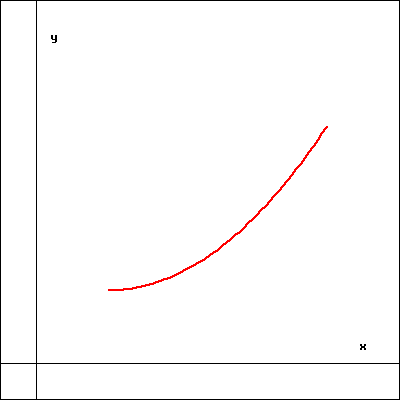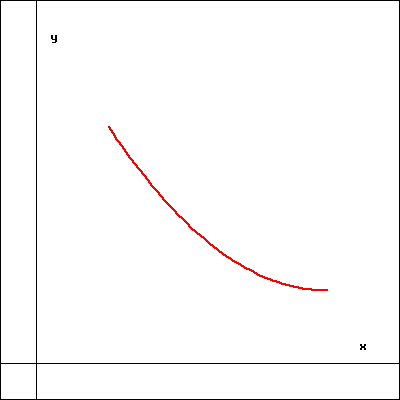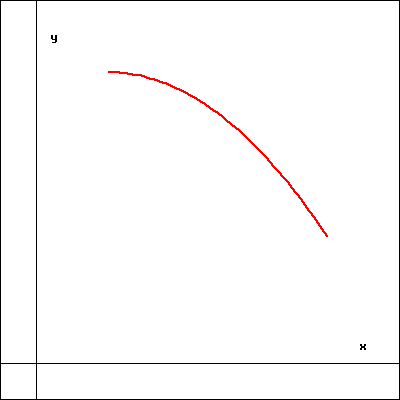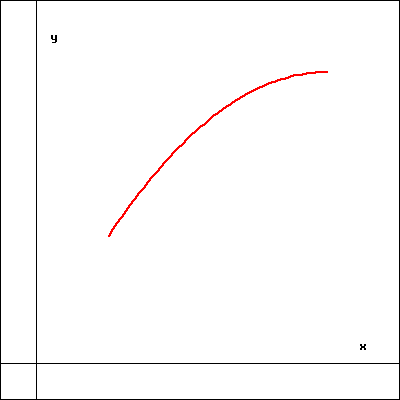Consider the function $f(x) = \frac{2 x^2}{x^2-9}$.

There is a vertical asymptote at: $x=$
NOTE: If there is more than one you can separate the values by a comma.

There a horizontal (or oblique) asymptote at $y =$
NOTE: This could be a function of x.

There is a y-intercept at $y=$ .

If $A$, $B$, and $C$ are critical points, then $f(x)$ can be broken up into the following unique intervals:
($-\infty,A$), ($A,B$), ($B,C$), ($C,\infty$).

$A =$
$B =$
$C =$

For each of the intervals listed below, select the graph best representing the shape of the function in that interval.

($-\infty,A$):
($A,B$):
($B,C$):
($C,\infty$):1 23 4

(Click on a graph to enlarge it.)

You can earn partial credit on this problem.